# Poincaré inequalities and the sharp maximal inequalities with ${L}^{\phi }$-norms for differential forms

## Abstract

This paper is concerned with the Poincaré inequalities and the sharp maximal inequalities for differential forms with ${L}^{\phi }$-norm, where φ satisfies nonstandard growth conditions. These results can be used to estimate the norms of classical operators and analyze integral properties of differential forms.

## 1 Introduction

In this paper, we consider the functional

$I\left(\mathrm{\Omega },v\right)={\int }_{\mathrm{\Omega }}\phi \left(|dv|\right)\phantom{\rule{0.2em}{0ex}}dx,$
(1)

where $\phi :\left[0,\mathrm{\infty }\right)\to \left[0,\mathrm{\infty }\right)$ is a Young function satisfying the nonstandard growth condition

$p\phi \left(t\right)\le t{\phi }^{\prime }\left(t\right)\le q\phi \left(t\right),\phantom{\rule{1em}{0ex}}1
(2)

This condition was first used in ; the author used this condition to get the higher integrability of the gradient of minimizers. We can find that the first inequality in (2) is equivalent to $\frac{\phi \left(t\right)}{{t}^{p}}$ that is increasing, and the second inequality in (2) is equivalent to ${\mathrm{△}}_{2}$-condition, i.e., for each $t>0$, $\phi \left(2t\right)\le K\phi \left(t\right)$, where $K>1$, and $\frac{\phi \left(t\right)}{{t}^{q}}$ is decreasing with t. Also, condition (2) implies that $\phi \left(t\right)$ satisfies

${c}_{1}{t}^{p}-{c}_{2}\le \phi \left(t\right)\le {c}_{3}\left({t}^{q}+1\right),$
(3)

but the exponents p, q in (2) may not be the best ones in order for (3) to hold. The following example can be found in , the convex function

satisfies (2) with $p=4-\sqrt{2}$ and $q=4+\sqrt{2}$ and satisfies (3) with $p=3$ and $q=5$. Moreover, if $4-\sqrt{2}, $\frac{\phi \left(t\right)}{{t}^{r}}$ is neither strictly increasing nor decreasing. Particularly, $\phi \left(t\right)={t}^{p}$ satisfies (2) because of $t{\phi }^{\prime }\left(t\right)=p\phi \left(t\right)$, and this makes inequalities with the norm ${\parallel \cdot \parallel }_{p}$ become a special case of Theorem 2.3, for more details see [1, 2].

We assume that Ω is a bounded convex domain in ${\mathbb{R}}^{n}$ ($n\ge 2$). Both B and σB are balls or cubes with the same center, $diam\left(\sigma B\right)=\sigma diam\left(B\right)$. $|E|$ is used to denote the n-dimensional Lebesgue measure of a set $E\subset {\mathbb{R}}^{n}$, and all integrals involved in this paper are the Lebesgue integrals. Let ${e}_{1},\dots ,{e}_{n}$ be the standard unit basis of ${\mathbb{R}}^{n}$. For $l=0,1,\dots ,n$, the linear space of l-vectors, spanned by the exterior products ${e}_{I}={e}_{{i}_{1}}\wedge {e}_{{i}_{2}}\wedge \cdots \wedge {e}_{{i}_{l}}$, corresponding to all ordered l-tuples $I=\left\{{i}_{i},{i}_{2},\dots ,{i}_{l}\right\}$, $1\le {i}_{1}<{i}_{2}<\cdots <{i}_{l}\le n$, is denoted by ${\mathrm{\Lambda }}^{l}={\mathrm{\Lambda }}^{l}\left({\mathbb{R}}^{n}\right)$. The Grassmann algebra $\mathrm{\Lambda }=\mathrm{\Lambda }\left({\mathbb{R}}^{n}\right)={⨁}_{l=0}^{n}{\mathrm{\Lambda }}^{l}\left({\mathbb{R}}^{n}\right)$ is a graded algebra with respect to the exterior products. A differential l-form ω on Ω is a Schwartz distribution on Ω with values in ${\mathrm{\Lambda }}^{l}\left({\mathbb{R}}^{n}\right)$, and it can be denoted by

$u\left(x\right)=\sum _{I}{u}_{I}\left(x\right)\phantom{\rule{0.2em}{0ex}}d{x}_{I}=\sum _{1\le {i}_{1}\le \cdots \le {i}_{l}\le n}{u}_{{i}_{1}{i}_{2}\cdots {i}_{l}}\left(x\right)\phantom{\rule{0.2em}{0ex}}d{x}_{{i}_{1}}\wedge \cdots \wedge d{x}_{{i}_{l}}.$

The exterior derivative $d:{\mathcal{D}}^{\prime }\left(\mathrm{\Omega },{\mathrm{\Lambda }}^{l}\right)\to {\mathcal{D}}^{\prime }\left(\mathrm{\Omega },{\mathrm{\Lambda }}^{l+1}\right)$ is expressed by

$du\left(x\right)=\sum _{j=1}^{n}\sum _{I}\frac{\partial {u}_{{i}_{1}\cdots {i}_{l}}}{\partial {x}_{j}}\phantom{\rule{0.2em}{0ex}}d{x}_{j}\wedge d{x}_{{i}_{1}}\wedge \cdots \wedge dx{i}_{l}.$

A differential l-form $u\in {\mathcal{D}}^{\prime }\left(\mathrm{\Omega },{\mathrm{\Lambda }}^{l}\right)$ is a closed form if $du=0$ in Ω. ${L}^{p}\left(\mathrm{\Omega },{\mathrm{\Lambda }}^{l}\right)$ is the space of l-forms $u\left(x\right)={\sum }_{I}{u}_{I}\left(x\right)\phantom{\rule{0.2em}{0ex}}d{x}_{I}$ with ${u}_{I}\in {L}^{p}\left(\mathrm{\Omega },\mathbb{R}\right)$ for all ordered l-tuples I, it is a Banach space endowed with the norm ${\parallel u\parallel }_{p,\mathrm{\Omega }}={\left({\int }_{\mathrm{\Omega }}{|u|}^{p}\phantom{\rule{0.2em}{0ex}}dx\right)}^{1/p}$. Similarly, ${W}^{1,p}\left(\mathrm{\Omega },{\mathrm{\Lambda }}^{l}\right)$ are those differential l-forms on Ω, whose coefficients are in ${W}^{1,p}\left(\mathrm{\Omega },\mathbb{R}\right)$, and it is a Banach space endowed with the norm ${\parallel u\parallel }_{1,p}={\parallel u\parallel }_{p}+{\parallel \mathrm{▽}u\parallel }_{p}$.

The following result was obtained in . For $y\in \mathrm{\Omega }$, there corresponds a linear operator ${K}_{y}:{C}^{\mathrm{\infty }}\left(\mathrm{\Omega },{\mathrm{\Lambda }}^{l}\right)\to {C}^{\mathrm{\infty }}\left(\mathrm{\Omega },{\mathrm{\Lambda }}^{l}\right)$ defined by $\left({K}_{y}u\right)\left(x;{\xi }_{1},\dots ,{\xi }_{l-1}\right)={\int }_{0}^{1}{t}^{l-1}u\left(tx+y-ty;x-y,{\xi }_{1},\dots ,{\xi }_{l-1}\right)\phantom{\rule{0.2em}{0ex}}dt$ and a decomposition $u=d\left({K}_{y}u\right)+{K}_{y}\left(du\right)$. A homotopy operator $T:{C}^{\mathrm{\infty }}\left(\mathrm{\Omega },{\mathrm{\Lambda }}^{l}\right)\to {C}^{\mathrm{\infty }}\left(\mathrm{\Omega },{\mathrm{\Lambda }}^{l}\right)$ is defined by averaging ${K}_{y}$ over all points y in Ω, i.e., $Tu={\int }_{\mathrm{\Omega }}\phi \left(y\right){K}_{y}u\phantom{\rule{0.2em}{0ex}}dy$, where $\phi \left(y\right)\in {C}_{0}^{\mathrm{\infty }}\left(\mathrm{\Omega }\right)$ is normalized by ${\int }_{\mathrm{\Omega }}\phi \left(y\right)\phantom{\rule{0.2em}{0ex}}dy=1$. Then, there is a decomposition $u=d\left(Tu\right)+T\left(du\right)$. The l-form ${u}_{\mathrm{\Omega }}\in {\mathcal{D}}^{\prime }\left(\mathrm{\Omega },{\mathrm{\Lambda }}^{l}\right)$ is defined by

${u}_{\mathrm{\Omega }}=\frac{1}{|\mathrm{\Omega }|}{\int }_{\mathrm{\Omega }}u\left(y\right)\phantom{\rule{0.2em}{0ex}}dy,\phantom{\rule{1em}{0ex}}l=0\phantom{\rule{1em}{0ex}}\text{and}\phantom{\rule{1em}{0ex}}{u}_{\mathrm{\Omega }}=d\left(Tu\right),\phantom{\rule{1em}{0ex}}l=1,2,\dots ,n$

for all $u\in {L}^{p}\left(\mathrm{\Omega },{\mathrm{\Lambda }}^{l}\right)$, $1\le p<\mathrm{\infty }$. For any differential forms, $u\in {L}_{\mathrm{loc}}^{s}\left(B,{\mathrm{\Lambda }}^{l}\right)$, $l=1,2,\dots ,n$, $1, we have

${\parallel \mathrm{▽}Tu\parallel }_{s,B}\le C|B|{\parallel u\parallel }_{s,B},\phantom{\rule{2em}{0ex}}{\parallel Tu\parallel }_{s,B}\le C|B|\left(diamB\right){\parallel u\parallel }_{s,B}.$
(4)

The rest of this paper is organized as follows. In Section 2, Poincaré inequalities for differential forms with Orlicz norm are obtained. In Section 3, the sharp maximal inequalities with Orlicz norms applied to k-quasiminimizer are obtained. In Section 4, using the methods that appeared in Section 2 and Section 3, we get some estimates of classical operators.

## 2 Poincaré inequalities

It is well known that the Poincaré inequality played an important role in studying the partial differential equations (PDEs) and the potential theory. Some versions of Poincaré inequalities for functions and differential forms with ${L}^{p}$-norm have been obtained, and in recent years, these inequalities for differential forms and operators with Luxemburg norm have been established, we refer reader to  and the references therein. First, we introduce some existing definitions and lemmas.

A continuously increasing function $\phi :\left[0,\mathrm{\infty }\right)\to \left[0,\mathrm{\infty }\right)$ with $\phi \left(0\right)=0$ is called an Orlicz function. The Orlicz space ${L}^{\phi }\left(\mathrm{\Omega }\right)$ consists of all measurable functions f on Ω satisfying ${\int }_{\mathrm{\Omega }}\phi \left(\frac{|f|}{\lambda }\right)\phantom{\rule{0.2em}{0ex}}dx\le \mathrm{\infty }$ for some $\lambda =\lambda \left(f\right)>0$, and it is equipped with the nonlinear Luxemburg functional

${\parallel f\parallel }_{\phi \left(\mathrm{\Omega }\right)}=inf\left\{\lambda >0:{\int }_{\mathrm{\Omega }}\phi \left(\frac{|f|}{\lambda }\right)\phantom{\rule{0.2em}{0ex}}dx\le 1\right\}.$
(5)

A convex Orlicz function φ is called a Young function. If φ is a Young function, then ${\parallel \cdot \parallel }_{\phi \left(\mathrm{\Omega }\right)}$ defines a norm in ${L}^{\phi }\left(\mathrm{\Omega }\right)$, which is called the Orlicz norm or Luxemburg norm, for more details see [5, 810, 12].

Lemma 2.1 

Let $u\in {\mathcal{D}}^{\prime }\left(B,{\mathrm{\Lambda }}^{l}\right)$ and $du\in {L}^{p}\left(B,{\mathrm{\Lambda }}^{l+1}\right)$. Then, $u-{u}_{B}$ is in ${L}^{\frac{np}{n-p}}\left(B,{\mathrm{\Lambda }}^{l}\right)$ and

${\left({\int }_{B}{|u-{u}_{B}|}^{\frac{np}{n-p}}\phantom{\rule{0.2em}{0ex}}dx\right)}^{\frac{n-p}{np}}\le c\left(n,p\right){\left({\int }_{B}|du|\phantom{\rule{0.2em}{0ex}}dx\right)}^{\frac{1}{p}}$
(6)

for B is a ball or cube in Ω, $l=0,1,\dots ,n$ and $1.

Lemma 2.2 

Suppose φ is a continuous function satisfying (2) with $q\left(n-p\right), $1. For any $t>0$, setting

$F\left(t\right)={\int }_{0}^{t}{\left(\frac{\phi \left({s}^{\frac{1}{q}}\right)}{s}\right)}^{\frac{n+q}{q}}\phantom{\rule{0.2em}{0ex}}ds,\phantom{\rule{2em}{0ex}}H\left(t\right)=\frac{{\left(\phi \left({t}^{\frac{1}{q}}\right)\right)}^{\frac{n+q}{q}}}{{t}^{\frac{n}{q}}}.$

Then, $F\left(t\right)$ is a concave function, and there exists a constant C, such that

$H\left(t\right)\le F\left(t\right)\le CH\left(t\right)\phantom{\rule{1em}{0ex}}\mathrm{\forall }t>0.$
(7)

Theorem 2.3 Suppose $u\in {\mathcal{D}}^{\prime }\left(\mathrm{\Omega },{\mathrm{\Lambda }}^{l}\right)$ and $du\in {L}^{p}\left(\mathrm{\Omega },{\mathrm{\Lambda }}^{l+1}\right)$, φ is a Young function satisfying (2) with $q\left(n-p\right), $1. Then, there exists a constant C, independent of u, such that

${\int }_{B}\phi \left(|u-{u}_{B}|\right)\phantom{\rule{0.2em}{0ex}}dx\le C{\int }_{B}\phi \left(|du|\right)\phantom{\rule{0.2em}{0ex}}dx,$
(8)

where B is a ball in Ω.

Proof Applying the Hölder inequality, we have(9)

Because of (7) and the concavity of F, which appeared in Lemma 2.2, (9) becomes(10)

If $1, by assumption, we have $q<\frac{np}{n-p}$. It follows from Lemma 2.1 that(11)

If $p\ge n$, note thatincreases with s and $\frac{ns}{n-s}\to \mathrm{\infty }$ as $s\to n$, thus, there exists $1<{p}_{0}, such that $q<\frac{n{p}_{0}}{n-{p}_{0}}$. Then, we have(12)

Combining (11) and (12), we get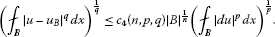(13)

Since φ is increasing and satisfies ${\mathrm{▵}}_{2}$-condition, (10) becomes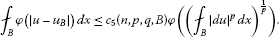(14)

Setting $\mathrm{\Phi }\left(t\right)={\int }_{0}^{t}\frac{\phi \left(s\right)}{s}\phantom{\rule{0.2em}{0ex}}dx$, it follows from (2) that $\frac{\phi \left(t\right)}{{t}^{q}}$ is decreasing with t, so

${\int }_{0}^{t}\frac{\phi \left(s\right)}{s}\phantom{\rule{0.2em}{0ex}}dx={\int }_{0}^{t}\frac{\phi \left(s\right)}{{s}^{q}}{s}^{q-1}\phantom{\rule{0.2em}{0ex}}dx\ge \frac{\phi \left(t\right)}{{t}^{q}}\cdot \frac{1}{q}{s}^{q}{|}_{0}^{t}=\frac{1}{q}\phi \left(t\right).$

Similarly, we have $\mathrm{\Phi }\left(t\right)\le \frac{1}{p}\phi \left(t\right)$. Thus,

$\frac{1}{q}\phi \left(t\right)\le \mathrm{\Phi }\left(t\right)\le \frac{1}{p}\phi \left(t\right).$
(15)

Let $\mathrm{\Psi }\left(t\right)=\mathrm{\Phi }\left({t}^{\frac{1}{p}}\right)$, ${\left(\mathrm{\Phi }\left({t}^{\frac{1}{p}}\right)\right)}^{\prime }=\frac{1}{p}\frac{\phi \left({t}^{\frac{1}{p}}\right)}{t}$ is increasing, so Ψ is a convex function.

For all $v\in {L}^{1}\left(B\right)$, by Jensen’s inequality, we get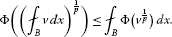Replace v with ${|du|}^{p}$, we have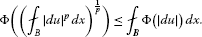(16)

Combining (15) and (16), (14) becomes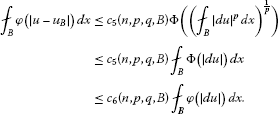This completes the proof. □

Since φ is a Young function satisfying ${\mathrm{▵}}_{2}$-condition, from the proof of Theorem 2.3, we have

${\int }_{B}\phi \left(\frac{|u-{u}_{B}|}{\lambda }\right)\phantom{\rule{0.2em}{0ex}}dx\le C{\int }_{B}\phi \left(\frac{|du|}{\lambda }\right)\phantom{\rule{0.2em}{0ex}}dx$

for any constant $\lambda >0$. According to (5), Poincaré-type inequality with Luxemburg norm

${\parallel u-{u}_{B}\parallel }_{\phi \left(B\right)}\le C{\parallel du\parallel }_{\phi \left(B\right)}$
(17)

holds under the conditions in Theorem 2.3.

Next, we extend the local Poincaré-type inequality into the global case in ${L}^{\varphi }$-averaging domain, which are extension of John domains and ${L}^{s}$-averaging domain, for more details see [5, 8, 9].

Definition 2.4 

Let ϕ be an increasing convex function on $\left[0,\mathrm{\infty }\right)$ with $\varphi \left(0\right)=0$, we call a proper subdomain $\mathrm{\Omega }\subset {\mathbb{R}}^{n}$ a ${L}^{\varphi }$-averaging domain if $|\mathrm{\Omega }|<\mathrm{\infty }$ and there exists a constant C, such that

${\int }_{\mathrm{\Omega }}\varphi \left(\tau |u-{u}_{{B}_{0}}|\right)\phantom{\rule{0.2em}{0ex}}dx\le C\underset{B\subset \mathrm{\Omega }}{sup}{\int }_{B}\varphi \left(\sigma |u-{u}_{B}|\right)\phantom{\rule{0.2em}{0ex}}dx$

for some ball ${B}_{0}\subset \mathrm{\Omega }$ and all x such that $\varphi \left(|u|\right)\in {L}_{\mathrm{loc}}^{I}\left(\mathrm{\Omega }\right)$, where τ, σ are constants with $0<\tau <\mathrm{\infty }$, $0<\sigma <\mathrm{\infty }$ and the supremum is over all balls $B\subset \mathrm{\Omega }$.

Similarly to the process of Theorem 3.2 in , we have the following theorem.

Theorem 2.5 Suppose that $u\in {\mathcal{D}}^{\prime }\left(\mathrm{\Omega },{\mathrm{\Lambda }}^{l}\right)$ and $du\in {L}^{p}\left(\mathrm{\Omega },{\mathrm{\Lambda }}^{l+1}\right)$, φ is a Young function satisfying (2) with $q\left(n-p\right), $1, Ω is any bounded ${L}^{\phi }$-averaging domain. Then, there exists a constant C, independent of u, such that

${\int }_{\mathrm{\Omega }}\phi \left(|u-{u}_{B}|\right)\phantom{\rule{0.2em}{0ex}}dx\le C{\int }_{\mathrm{\Omega }}\phi \left(|du|\right)\phantom{\rule{0.2em}{0ex}}dx,$
(18)

where B is a ball or cube in Ω.

Choosing $\phi \left(t\right)={t}^{p}{log}^{\alpha }\left(e+t\right)$, $p\phi \left(t\right)\le t{\phi }^{\prime }\left(t\right)\le \left(p+\alpha \right)\phi \left(t\right)$ can be checked easily, from Theorem 2.5, we can get Poincaré inequality with ${L}^{p}\left({log}^{\alpha }L\right)$-norm.

Corollary 2.6 Suppose that u is a smooth differential form, $\phi \left(t\right)={t}^{p}{log}^{\alpha }\left(e+t\right)$, $1. Ω is a bounded ${L}^{\phi }$-averaging domain. Then, there exists a constant C, independent of u, such that

${\int }_{\mathrm{\Omega }}{|u-{u}_{B}|}^{p}{log}^{\alpha }\left(|u-{u}_{B}|\right)\phantom{\rule{0.2em}{0ex}}dx\le C{\int }_{\mathrm{\Omega }}{|du|}^{p}{log}^{\alpha }\left(|du|\right)\phantom{\rule{0.2em}{0ex}}dx.$
(19)

## 3 Inequalities for the sharp maximal operator applied to minimizers

Let ${B}_{0}$ be a fixed open ball in a bounded domain Ω. For differential form $u\in {L}^{s}\left({B}_{0},{\mathrm{\Lambda }}^{l}\right)$, $1\le s<\mathrm{\infty }$, $l=0,1,\dots ,n$, the Hardy-Littlewood maximal operator of order s is defined by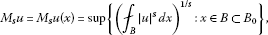where the supremum is taken over all parallel open subcubes B of ${B}_{0}$ containing the point x. Write ${M}_{1}u\left(x\right)=Mu\left(x\right)$ if $s=1$. Similarly, the sharp maximal operator ${M}_{s}^{\mathrm{♯}}$ is defined byWe say that a differential form $u\in {W}^{1,1}\left(\mathrm{\Omega },{\mathrm{\Lambda }}^{l}\right)$ is a k-quasiminimizer for the functional

$I\left(\mathrm{\Omega },u\right)={\int }_{\mathrm{\Omega }}\phi \left(|du|\right)\phantom{\rule{0.2em}{0ex}}dx$
(20)

if and only if for every $\psi \in {W}^{1,1}\left(\mathrm{\Omega },{\mathrm{\Lambda }}^{l}\right)$ with compact support

$I\left(supp\psi ;u\right)\le kI\left(supp\psi ;u+\psi \right),$
(21)

where $k>1$ is a constant.

The following lemmas will be used in this section.

Lemma 3.1 

If ${B}_{0}$ is a cube in Ω, $f\in {L}^{s}\left({B}_{0}\right)$, $f\ge 0$. Then, for any $t>0$,

$|\left\{x\in {B}_{0}:{M}_{s}f\left(x\right)>t\right\}|\le \frac{c\left(n\right)}{{t}^{s}}{\int }_{\left\{f>t/2\right\}}{|f\left(x\right)|}^{s}\phantom{\rule{0.2em}{0ex}}dx.$
(22)

Lemma 3.2 

Let u be a k-quasiminimizer for the functional (20), and let φ be a Young function satisfying ${\mathrm{△}}_{2}$-condition. Then, for any ball ${B}_{R}\subset \mathrm{\Omega }$ with radius R, there exists a constant C, independent of u such that

${\int }_{{B}_{R/2}}\phi \left(|du|\right)\phantom{\rule{0.2em}{0ex}}dx\le C{\int }_{{B}_{R}}\phi \left(\frac{|u-c|}{R}\right)\phantom{\rule{0.2em}{0ex}}dx,$
(23)

where c is any closed form.

The main result of this section is the following theorem.

Theorem 3.3 Suppose u is a k-quasiminimizer for the functional (20), and φ is a Young function satisfying (2) with $q\left(n-p\right), $1, ${M}_{s}^{\mathrm{♯}}$ is the sharp maximal operator, ${B}_{0}\subset \mathrm{\Omega }$ is a ball satisfying $2{B}_{0}\subset \mathrm{\Omega }$. Then, for $1\le s, there exists a constant C, independent of u such that

${\int }_{{B}_{0}}\phi \left(|{M}_{s}^{\mathrm{♯}}u|\right)\phantom{\rule{0.2em}{0ex}}dx\le C{\int }_{2{B}_{0}}\phi \left(|u-c|\right)\phantom{\rule{0.2em}{0ex}}dx,$
(24)

where c is a closed form.

Proof Similarly to (10), we obtainDenote $f=|u-{u}_{B}|$, then ${M}_{s}f={M}_{s}^{\mathrm{♯}}u$. It follows from the standard representation theorem and Lemma 3.1 that,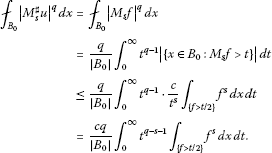(25)

By Fubini’s theorem, (25) becomes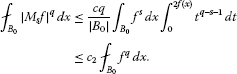(26)

That is,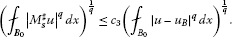For similar discussion of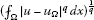in Theorem 2.3, we have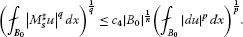Then,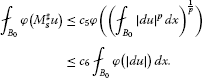(27)

Since φ satisfying ${\mathrm{△}}_{2}$-condition, from Lemma 3.2, we getwhere c is a closed form. This completes the proof. □

Similarly to (17), the sharp maximal inequality with Luxemburg norm

${\parallel {M}_{s}^{\mathrm{♯}}u\parallel }_{\phi \left({B}_{0}\right)}\le C{\parallel u-c\parallel }_{\phi \left({B}_{0}\right)}$
(28)

holds under the conditions described in Theorem 3.3.

## 4 Application

As an application, we develop some estimates for homotopy operator T, Green’s operator G and the composition of the operators $T\circ G$. Inequalities for the other class operators and composition operators can be obtained similarly.

Let . The harmonic l-fields are defined by . The orthogonal complement of in ${L}^{1}\left(\mathrm{\Omega },{\mathrm{\Lambda }}^{l}\right)$ is defined by . Then, the Green’s operator G is defined as $G:{C}^{\mathrm{\infty }}\left(\mathrm{\Omega },{\mathrm{\Lambda }}^{l}\right)\to {\mathcal{H}}^{\perp }\cap {C}^{\mathrm{\infty }}\left(\mathrm{\Omega },{\mathrm{\Lambda }}^{l}\right)$ by assigning $G\left(u\right)$ to be the unique element of ${\mathcal{H}}^{\perp }\cap {C}^{\mathrm{\infty }}\left(\mathrm{\Omega },{\mathrm{\Lambda }}^{l}\right)$ satisfying Poisson’s equation $\mathrm{△}G\left(u\right)=u-\mathcal{H}\left(u\right)$, where is the harmonic projection operator that maps ${C}^{\mathrm{\infty }}\left(\mathrm{\Omega },{\mathrm{\Lambda }}^{l}\right)$ on , so that $\mathcal{H}\left(u\right)$ is the harmonic of u. See  for more properties of Green’s operator.

Next, we prove the estimate for homotopy operator T.

Theorem 4.1 Suppose that u is a smooth differential form, φ is a Young function satisfying (2) with $q\left(n-p\right), $1. T is a homotopy operator. Then, there exists a constant C, independent of u, such that

${\int }_{B}\phi \left(|T\left(u\right)-{\left(T\left(u\right)\right)}_{B}|\right)\phantom{\rule{0.2em}{0ex}}dx\le C{\int }_{B}\phi \left(|u|\right)\phantom{\rule{0.2em}{0ex}}dx$
(29)

for all balls $B\subset \mathrm{\Omega }$.

Proof Since $|dv|\le c|\mathrm{▽}v|$ holds for each differential form v, combining (4) and the similar process of (10), we have

$\begin{array}{rcl}{\int }_{B}\phi \left(|T\left(u\right)-{\left(T\left(u\right)\right)}_{B}|\right)\phantom{\rule{0.2em}{0ex}}dx& \le & {c}_{1}\phi {\left(\left({\int }_{B}{|T\left(u\right)-{\left(T\left(u\right)\right)}_{B}|}^{q}\right)\phantom{\rule{0.2em}{0ex}}dx\right)}^{\frac{1}{q}}\\ \le & {c}_{1}\phi \left({c}_{2}{\left({\int }_{B}{|d\left(T\left(u\right)\right)|}^{p}\phantom{\rule{0.2em}{0ex}}dx\right)}^{\frac{1}{p}}\right)\\ \le & {c}_{3}\phi \left({\left({\int }_{B}{|\mathrm{▽}\left(T\left(u\right)\right)|}^{p}\phantom{\rule{0.2em}{0ex}}dx\right)}^{\frac{1}{p}}\right)\\ \le & {c}_{3}\phi \left({c}_{4}{\left({\int }_{B}{|u|}^{p}\phantom{\rule{0.2em}{0ex}}dx\right)}^{\frac{1}{p}}\right)\\ \le & {c}_{5}{\int }_{B}\phi \left(|u|\right)\phantom{\rule{0.2em}{0ex}}dx.\end{array}$

□

For Green’s operator, we have the following estimate for Green’s operator applied to k-quasiminimizer.

Theorem 4.2 Suppose that the smooth differential form u is a k-quasiminimizer for the functional (20), and φ is a Young function satisfying (2) with $q\left(n-p\right), $1, G is a Green’s operator. Then, for each ball $B\subset \mathrm{\Omega }$ with $2B\subset \mathrm{\Omega }$, there exists a constant C, independent of u, such that

${\int }_{B}\phi \left(|G\left(u\right)-{\left(G\left(u\right)\right)}_{B}|\right)\phantom{\rule{0.2em}{0ex}}dx\le C{\int }_{\sigma B}\phi \left(|u-c|\right)\phantom{\rule{0.2em}{0ex}}dx,$
(30)

where $\sigma >1$ is a constant, c is a closed form.

Proof Since

${\parallel G\left(u\right)\parallel }_{p,B}\le c{\parallel u\parallel }_{p,B}$
(31)

holds for differential form u and G commutes with differential operator d, we have

$\begin{array}{rcl}{\int }_{B}\phi \left(|G\left(u\right)-{\left(G\left(u\right)\right)}_{B}|\right)\phantom{\rule{0.2em}{0ex}}dx& \le & {c}_{1}\phi \left({\left({\int }_{B}{|G\left(u\right)-{\left(G\left(u\right)\right)}_{B}|}^{q}\phantom{\rule{0.2em}{0ex}}dx\right)}^{\frac{1}{q}}\right)\\ \le & {c}_{1}\phi \left({c}_{2}{\left({\int }_{B}{|G\left(u\right)-{\left(G\left(u\right)\right)}_{B}|}^{p}\phantom{\rule{0.2em}{0ex}}dx\right)}^{\frac{1}{p}}\right)\\ \le & {c}_{3}\phi \left({\left({\int }_{B}{|d\left(G\left(u\right)\right)|}^{p}\phantom{\rule{0.2em}{0ex}}dx\right)}^{\frac{1}{p}}\right)\\ =& {c}_{3}\phi \left({c}_{4}{\left({\int }_{B}{|G\left(du\right)|}^{p}\phantom{\rule{0.2em}{0ex}}dx\right)}^{\frac{1}{p}}\right)\\ \le & {c}_{5}{\int }_{B}\phi \left(|du|\right)\phantom{\rule{0.2em}{0ex}}dx.\end{array}$

It follows from Lemma 3.2 that

${\int }_{B}\phi \left(|G\left(u\right)-{\left(G\left(u\right)\right)}_{B}|\right)\phantom{\rule{0.2em}{0ex}}dx\le {c}_{6}{\int }_{\sigma B}\phi \left(|u-c|\right)\phantom{\rule{0.2em}{0ex}}dx.$

This completes the proof of Theorem 4.2. □

Corollary 4.3 Suppose that u is a smooth differential form, φ is a Young function satisfying (2) and $q\left(n-p\right), $1. When T is a homotopy operator and G is a Green’s operator, there exists a constant C, independent of u such that

${\int }_{B}\phi \left(|T\left(G\left(u\right)\right)-{\left(T\left(G\left(u\right)\right)\right)}_{B}|\right)\phantom{\rule{0.2em}{0ex}}dx\le C{\int }_{B}\phi \left(|u|\right)\phantom{\rule{0.2em}{0ex}}dx$
(32)

for all balls $B\subset \mathrm{\Omega }$.

Proof From (31) and Theorem 4.1, we have

$\begin{array}{rcl}{\int }_{B}\phi \left(|T\left(G\left(u\right)\right)-{\left(T\left(G\left(u\right)\right)\right)}_{B}|\right)\phantom{\rule{0.2em}{0ex}}dx& \le & {c}_{1}\phi \left({\left({\int }_{B}{|G\left(u\right)|}^{p}\phantom{\rule{0.2em}{0ex}}dx\right)}^{\frac{1}{p}}\right)\\ \le & {c}_{1}\phi \left({c}_{2}{\left({\int }_{B}{|u|}^{p}\phantom{\rule{0.2em}{0ex}}dx\right)}^{\frac{1}{p}}\right)\\ \le & {c}_{3}{\int }_{B}\phi \left(|u|\right)\phantom{\rule{0.2em}{0ex}}dx.\end{array}$

This ends the proof of Corollary 4.3. □

Remark 4.4 In 2004, Buckley and Keoskela first introduced a function class $G\left(p,q,C\right)$ in . After that, some mathematicians devoted themselves to study the inequalities with ${L}^{\psi }$-norm for differential form and operators, where ψ lies in $G\left(p,q,C\right)$. We find that ${t}^{p}$ and ${t}^{p}{log}^{\alpha }\left(e+t\right)$ are both of function class $G\left(p,q,C\right)$ and satisfying condition (2) in this paper. But it is still open which of the conditions of function class $G\left(p,q,C\right)$ and (2) is stronger, or they are not inclusive of each other.

## References

1. Fusco N, Sbordone C: High integrability of gradient of minimizers of functionals with nonstandard growth conditions. Commun. Pure Appl. Math. 1990, 43: 673–683. 10.1002/cpa.3160430505

2. Sbordone C: Maximal inequalities and applications to regularity problem in the calculus of variations. Calculus of Variations, Applications and Computations 1994.

3. Iwaniec T, Lutoborski A: Integral estimates for null Lagrangians. Arch. Ration. Mech. Anal. 1993, 125: 25–79. 10.1007/BF00411477

4. Agarwal RP, Ding S, Nolder CA: Inequalities for Differential Forms. Springer, New York; 2009.

5. Ding S, Zhu J:Poincaré-type inequalities for the homotopy operator with ${L}^{\phi }\left(\mathrm{\Omega }\right)$-norms. Nonlinear Anal. 2011, 74: 3728–3735. 10.1016/j.na.2011.03.018

6. Ding S, Liu B: Generalized Poincaré inequalities for solutions to the A -harmonic equation in certain domains. J. Math. Anal. Appl. 2000, 252: 538–548. 10.1006/jmaa.2000.6951

7. Nolder CA: Hardy-Littlewood theorems for A -harmonic tensors. Ill. J. Math. 1999, 43: 613–631.

8. Ding S:${L}^{\phi }$-Averaging domain and Poincaré inequalities with Orlicz norm. Nonlinear Anal. 2010, 73: 256–265. 10.1016/j.na.2010.03.018

9. Xing Y:Poincaré inequalities with Luxemburg norms in ${L}^{\phi }\left(m\right)$-averaging domains. J. Inequal. Appl. 2010., 2010: Article ID 24715

10. Agarwal RP, Ding S: Global Caccioppoli-type and Poincaré inequalities with Orlicz norms. J. Inequal. Appl. 2010., 2010: Article ID 727954

11. Ling Y, Bao G: Some local Poincaré inequalities for the composition of the sharp maximal operator and the Green’s operator. Comput. Math. Appl. 2012, 63: 720–727.

12. Agarwal RP, Ding S: Inequalities for Green’s operator applied to the minimizers. J. Inequal. Appl. 2011., 2011: Article ID 66

13. Wang Y, Wu C: Sobolev imbedding theorems and Poincaré inequalities for Green’s operator on solutions of the non-homogeneous A -harmonic equation. Comput. Math. Appl. 2004, 47: 1545–1554.

14. Bojarski B, Iwaniec T:Analytical foundations of the theory of quasiconformal mappings in ${\mathbb{R}}^{n}$. Ann. Acad. Sci. Fenn. 1983, 8: 257–324.

15. Buckley SM, Koskela P: Orlicz-Hardy inequalities. Ill. J. Math. 2004, 48: 787–802.

## Acknowledgements

The authors would like to thank the anonymous referees for their time and thoughtful suggestions. The research is supported by the National Science Foundation of China (# 11071048).

## Author information

Authors

### Corresponding authors

Correspondence to Yueming Lu or Gejun Bao.

### Competing interests

The authors declare that they have no competing interests.

### Authors’ contributions

All authors contributed equally in this paper. They read and approved the final manuscript.

## Rights and permissions

Reprints and Permissions

Lu, Y., Bao, G. Poincaré inequalities and the sharp maximal inequalities with ${L}^{\phi }$-norms for differential forms. J Inequal Appl 2013, 400 (2013). https://doi.org/10.1186/1029-242X-2013-400

• Accepted:

• Published:

• DOI: https://doi.org/10.1186/1029-242X-2013-400

### Keywords

• Poincaré inequalities
• sharp maximal inequalities
• nonstandard growth conditions
• differential forms